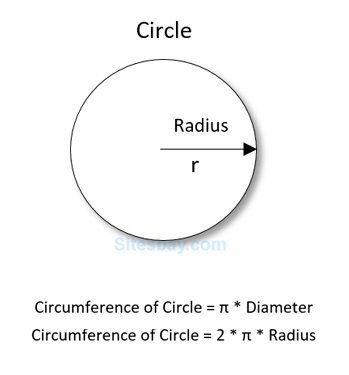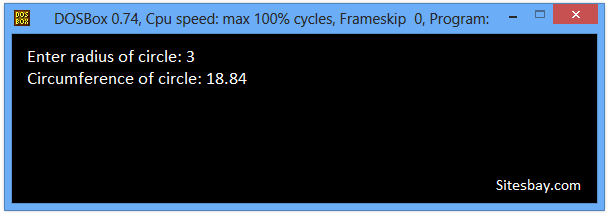# Find Circumference of circle in C++

## Find Circumference of Circle Program in C++

In below C++ program, we will calculate circumference and area of circle given radius of the circle. The area of circle is the amount of two-dimensional space taken up by a circle. We can calculate the area of a circle if you know its radius. Area of circle is measured in square units.

Circumference of circle is the length of the curved line which defines the boundary of a circle. The perimeter of a circle is called the circumference.## Formula

```Circumference or Circle = PI X Diameter

Where, Where PI is a constant which is equal to 22/7 or 3.141(approx)
```

## Program to Find Circumference of Circle

```#include<iostream.h>
#include<conio.h>
#define PI 3.141

void main()
{
clrscr();
// Circumference of Circle = 2 X PI x Radius
cout<<"Circumference of circle: "<<circumference;

getch();
}
```

## Output

```Enter radius of circle: 3
Circumference of circle: 18.84
```## Pure VPN Privide Lowest Price VPN Just @ \$1.65. Per Month with Non Detected IP Lowest Price Non Detected IP VPN